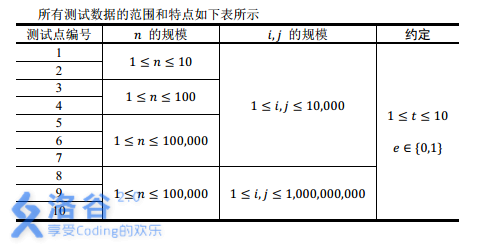o
osc_z1hvg4cu

## 输入输出样例

``````2
2
1 2 1
1 2 0
2
1 2 1
2 1 1``````

``````NO
YES
``````

``````2
3
1 2 1
2 3 1
3 1 1
4
1 2 1
2 3 1
3 4 1
1 4 0
``````

``````YES
NO``````

## 说明

【样例解释1】

【样例说明2】

【数据范围】【时限2s，内存512M】

分析：一眼可知是并查集，问题就在离散化，数据范围肯定不能直接上。但是蒟蒻并不擅长离散化，一开始还好感人地打了个hash，搞了半天结果屏幕上wa声一片……然后翻了翻大佬们的博客，发现。。。模个数就行了（O.o）。。好吧，是我太蒻了。

Code：

``````#include<cstdio>
#include<cstring>
#include<cstdlib>
#include<cmath>
#include<iostream>
#include<iomanip>
#include<algorithm>
using namespace std;
const int mod=528419;
const int N=1e5+7;
int n,T,cnt,fa[mod+7],rank[mod+7];
struct Ques{int x,y;}a[N];
{
char ch=getchar();int num=0;bool flag=false;
while(ch<'0'||ch>'9'){if(ch=='-')flag=true;ch=getchar();}
while(ch>='0'&&ch<='9'){num=num*10+ch-'0';ch=getchar();}
return flag?-num:num;
}
{
cnt=0;
for(int i=1;i<=mod;i++)fa[i]=i;
for(int i=1;i<=mod;i++)rank[i]=1;
}
inline int find(int x)
{return fa[x]==x?x:fa[x]=find(fa[x]);}
inline void merge(int x,int y)
{
if(rank[x]<rank[y]){
fa[x]=y;}
else{
fa[y]=x;
if(rank[x]==rank[y])rank[x]++;}
}
inline int work()
{
for(int i=1;i<=n;i++){
x%=mod;y%=mod;
if(e)merge(find(x),find(y));
else{a[++cnt].x=x;a[cnt].y=y;}
}
bool flag=true;
for(int i=1;i<=cnt;i++)
if(find(a[i].x)==find(a[i].y))
{flag=false;break;}
if(flag)puts("YES");
else puts("NO");
}
int main()
{
while(T--){
return 0;
}``````

o

### osc_z1hvg4cu#### 暂无文章

Vue-插槽slot/具名插槽

2分钟前
0
0

20分钟前
24
0

20
0

17
0

16
0